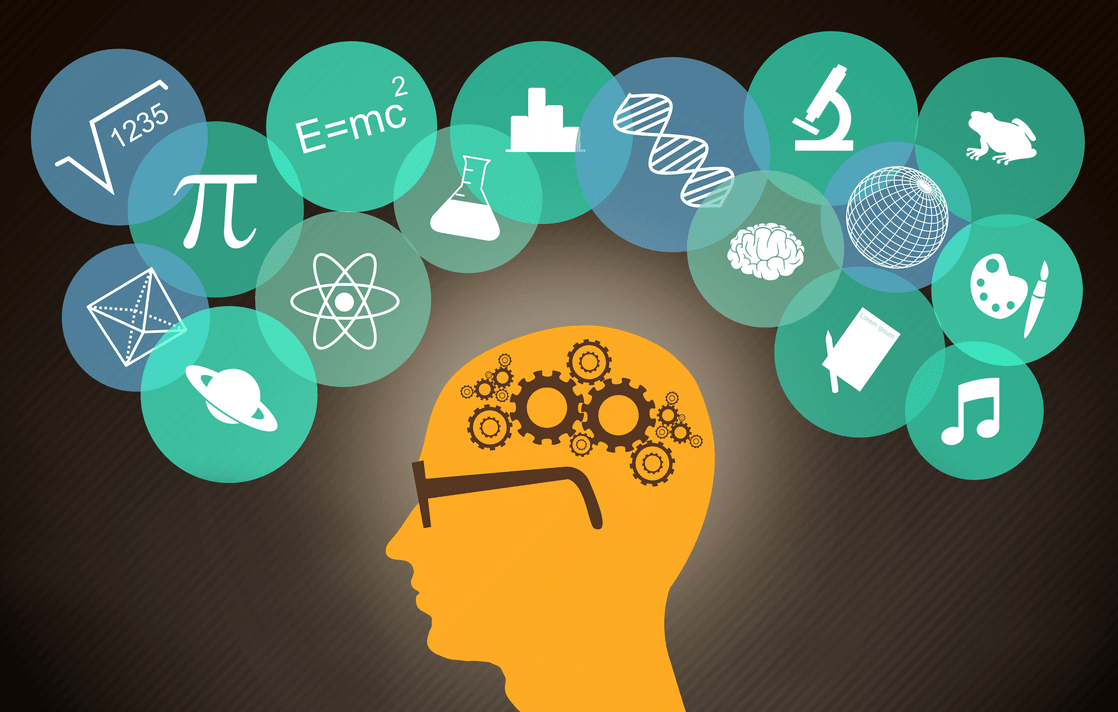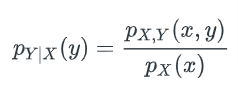# How Much Math Do You Need to Know in Data Science?

## Overview

Mathematics is one subject that scares a lot of people interested in data science and machine learning. In fact, math is the topic of numerous questions we get asked by early-career data scientists. They all seem to ask a version of:

### Is Math needed for data science?

The short answer is that it’s yes, it is important. And if you’re interested in a data science career with no math background, you’ll likely struggle. But the good news is that the most fundamental mathematical concepts in data science can be learned (even if you don’t have much math experience).

But we wanted to answer that question for our readers - do I need to know math for data science? Is it a requirement in becoming a data scientist? - and provide an overview of some of the most useful mathematical concepts practitioners use.

The bottom line is this: Math - or more broadly, the quantitative reasoning skills a math background provides - is essential for many day-to-day job tasks.

## What Math Do Data Scientists Use?How much math is needed in the field of data science? A wide range of mathematical concepts is put into play. But if you’re starting from scratch, you should focus your studies on three core areas, the so-called Big Three.

This includes: Linear algebra, calculus, and most importantly, statistics and probability.

### 1. Statistics

Statistics is used nearly every day by data scientists. In fact, the majority of data science interviews for FAANG jobs will ask statistics questions.

In data science, statistics is used for trend-spotting and forecasting, predictive modeling, and hypothesis testing, to name a few applications. For example, if a product manager asked you to forecast sales, you might turn to a concept like regression analysis. Core concepts to know include:

• Normal Distribution
• Mean, Median and More
• Standard Deviation/Variance
• Hypothesis testing, A/B testing, and confidence intervals
• Probability distributions (Binomial, Poisson, normal)
• P-Value
• Basic probability
• Data summaries and descriptive statistics

### 2. Linear Algebra

One of the fundamental branches of math, linear algebra, applies to many data science processes. For example, linear algebra is essential for understanding many algorithms and prediction models. With linear algebra, it’s important to have a strong grasp of the fundamentals (although unlike stats, basic knowledge might be all that’s necessary. Core concepts to know include:

• Graphing functions
• Multiplying matrices, matrix inverse, transpose of a matrix
• Scalar multiplication, linear transformation
• Matrix factorization, Gaussian elimination

### 3. Calculus

Calculus concepts are used for several key data science techniques. For example, backpropagation algorithms used to train neural networks are typically based on the chain rule of calculus. Core concepts to know include:

• Maxima and minima
• Functions: Single variable and multiple variable, beta and gamma functions
• Mean value theorem, fundamental theorem
• Product and chain rule
• Taylor’s series, infinite series summation

## How Is Math Used in Data Science?

At the risk of being overly broad, a data scientist’s key job role is to mine, analyze and interpret data. And at each stage, math plays a role.

Really, a good way to think about how math is used is to think about some of the core techniques data scientists use: Clustering, regression and classification. Math forms the basis of all of these techniques:

1. Clustering – Clustering is all about determining how data should be grouped. And there’s a lot of statistics and calculus behind these techniques like the K-means algorithm and mean-shift clustering.

2. Regression – Regression techniques are used by data scientists to make data-driven predictions. Concepts like linear regressions and multivariate regressions - from both linear algebra and stats - come in handy.

3. Classification – Classification techniques to sort data are built on math. For example, K-nearest neighbor classification is built around calculus formulas and linear algebra.

In interviews and on the job, you should be able to identify which of these techniques applies to a problem, given the characteristics of the data.

## What Types of Data Science Questions Does Math Help Us Solve?

Really, you can apply math to a variety of data science questions. These are just a few of the possibilities:

• Anomaly Detection – You might be asked: Is an anomaly random or out-of-the-ordinary? Clustering techniques like mean-shift (from calculus) might be applied to answer it.
• A/B testing – A/B testing incorporates a lot of stats concepts, like confidence intervals, causation and correlation, and experimental design.
• Algorithm design - Discrete math and calculus are helpful for understanding algorithm basics.
• Linear modeling - A basic algebra concept is useful for regression and classification techniques.
• Time series - Numerous product and business metrics are in a time series. A strong understanding is helpful for data analysis.
• Machine learning – Statistics forms the basis of machine learning.
• Quantitative reasoning - The quantitative problem-solving skills you gain in math come in handy when solving BI questions like why a trend is occurring.

## Sample Data Science Math Questions

In nearly every data science interview, you’ll be asked math questions. Statistics are the most common, but calculus- and linear algebra-based questions do get asked. The key is prep. Practice as many sample interview questions as you can.

### 5. Let’s say that you’re drawing N cards from a deck of 52 cards. Compute the probability that you will get a pair from your hand of N cards.

Let’s calculate it out.

If we draw N cards from a deck of 52, the probability that the first card is a not a pair is 100%, given that you need at least two cards to make a pair.

The probability that the second card is a new card ranking is 4851. How did we compute that?

### 6. Let’s say you have a function that outputs a random integer between a minimum value, N, and maximum value, M.

Now let’s say we take the output from the random integer function and place it into another random function as the max value with the same min value N.

1. What would the distribution of the samples look like?

2. What would be the expected value?## Bottom Line: Math Helps You Answer the ‘Why’

Today, data scientists have a lot of tools at their disposal: pre-packaged algorithms, libraries and packages. But a challenge if you don’t have strong math fundamentals is understanding why these models work. Without understanding the why, it’s difficult - if not impossible - to improve upon them.

And improving upon methodologies and inventing your own is the fastest way to become a data scientist. With a strong math background, for example, you’d have a basis for dissecting new methodologies, quickly understanding why and how they work, and using those methods in your own solutions.

Without math, you can certainly find jobs in data science and perform basic tasks, like decision-tree classification, but the advanced methods will likely remain elusive.